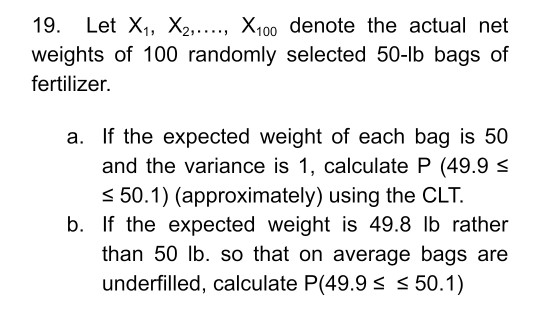# Question 19. Let X1, X2,..., X100 denote the actual net weights of 100 randomly selected 50-1b bags of fertilizer. a. If the expected weight of each bag is 50 and the variance is 1, calculate P (49.9 S 550.1) (approximately) using the CLT. b. If the expected weight is 49.8 lb rather than 50 lb. so that on average bags are underfilled, calculate P(49.9 S S 50.1)PDQRKK The Asker · Probability and StatisticsTranscribed Image Text: 19. Let X1, X2,..., X100 denote the actual net weights of 100 randomly selected 50-1b bags of fertilizer. a. If the expected weight of each bag is 50 and the variance is 1, calculate P (49.9 S 550.1) (approximately) using the CLT. b. If the expected weight is 49.8 lb rather than 50 lb. so that on average bags are underfilled, calculate P(49.9 S S 50.1)
More
Transcribed Image Text: 19. Let X1, X2,..., X100 denote the actual net weights of 100 randomly selected 50-1b bags of fertilizer. a. If the expected weight of each bag is 50 and the variance is 1, calculate P (49.9 S 550.1) (approximately) using the CLT. b. If the expected weight is 49.8 lb rather than 50 lb. so that on average bags are underfilled, calculate P(49.9 S S 50.1)